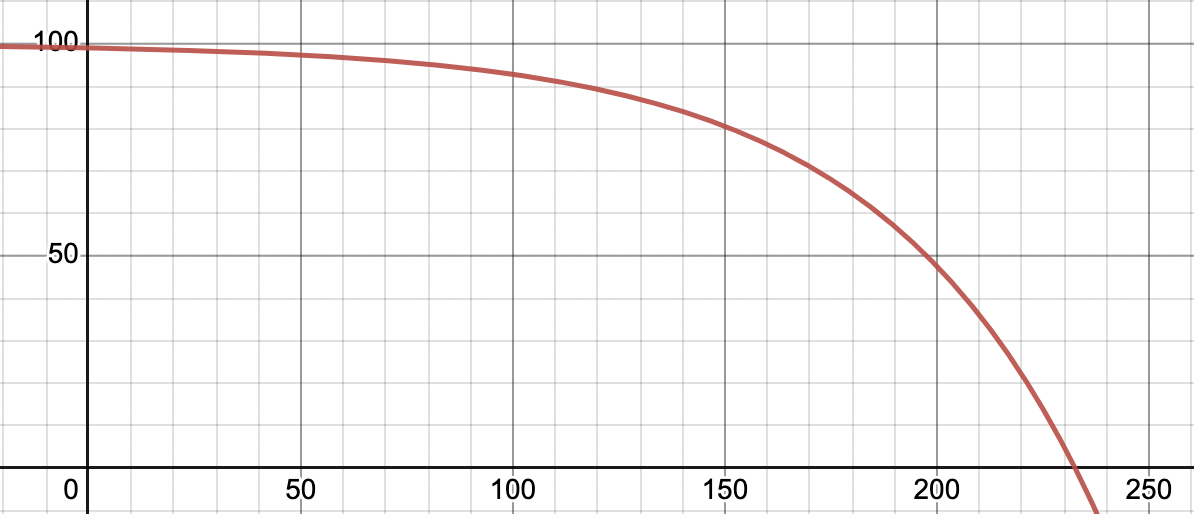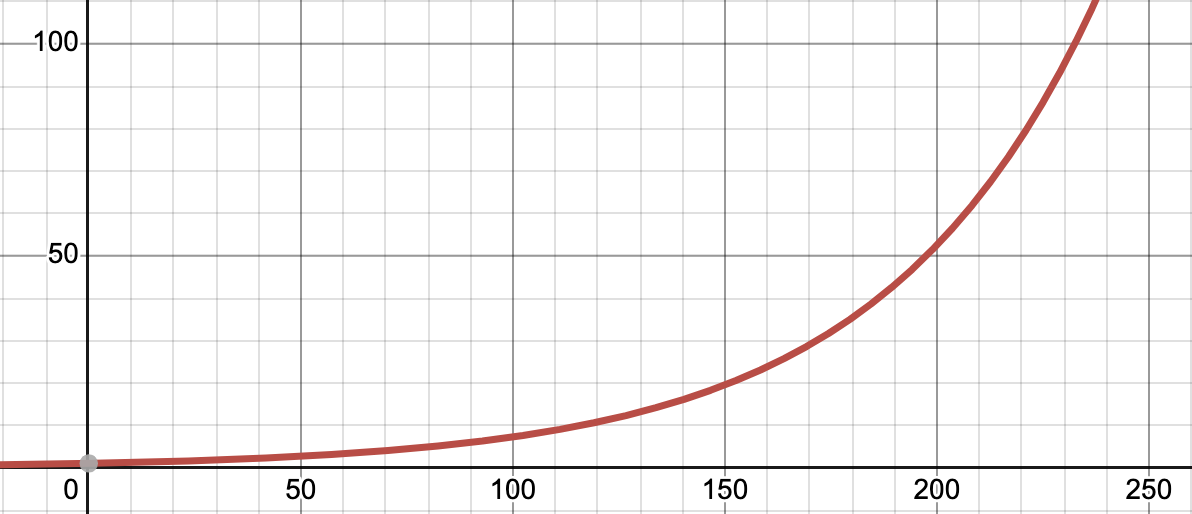# Reducing programming complexity is a delusion

01 February 2023

Every day, a new article has been published to show how to reduce programming complexity in your code. Best practices, new methods, and techniques are in every corner. But we are not questioning what it means, or even if it is really possible.

I claim that reducing programming complexity is a delusion. Let's talk about it.

## Boundaries

I must clarify some points before deep diving in.

I am not saying that the code complexity is not reducible at all. I accept the truth that we -programmers- are making mistakes and creating more complex code than we need. But I refuse many solution or methods out there that claims that they are reducing complexity.

## The source of the complexity

Every code that we produce is complex at some level. We can't talk about a code with zero complexity. There will be always complexity in our code because every piece of code is a solution to a problem. The complexity comes from the problem. The code would be complex if the problem is getting complex. There is no escape from this reality.

Every business logic, every customer request, every algorithm, and every edge case brings more complexity.

## Measuring the complexity

Let's assume that we have a code like the following example, and ignore what it is for. Just focus on the complexity value. We are going to reduce this function's complexity later.

``````function doSomeComplexTask(data) {
if (data.param1 === data.param2) {
param1: null,
param2: 666,
param3,
param5,
param4,
});
}

if (data.param1 === "is-disabled") {
data.param1 = data.param4;

if (data.param5 === "is-active") {
data.param2 = data.param4;
}

if (data.param1 == data.param2) {
data.param3 = data.param1 + data.param2 + data.param3;
} else if (data.param4 === "customer") {
data.param3 = data.param5 - data.param1;
} else {
param1: null,
param2: null,
param3: null,
param5: null,
param4: null,
});
}
}

}
``````

Cyclomatic complexity number for this function is 6.

Now we are going to split this function into little pieces to reduce the complexity.

``````function doSomeComplexTask(data) {
if (data.param1 === data.param2) {
param1: null,
param2: 666,
param3,
param5,
param4,
});
}

checkSpecialCase(data);

}
``````

We extracted the piece of code from the main function to another function. Now the complexity value is 2.

The new function, `checkSpecialCase`, would be like the following code.

``````function checkSpecialCase(data) {
if (data.param1 === "is-disabled") {
data.param1 = data.param4;

if (data.param5 === "is-active") {
data.param2 = data.param4;
}

if (data.param1 == data.param2) {
data.param3 = data.param1 + data.param2 + data.param3;
} else if (data.param4 === "customer") {
data.param3 = data.param5 - data.param1;
} else {
param1: null,
param2: null,
param3: null,
param5: null,
param4: null,
});
}
}
}
``````

The complexity value of this function would be 5. This is a simple demonstration of how we can reduce the complexity of our code. In almost every case, having two maintainable functions (2, 5) is better than a complicated function (6).

## The missing point

We are applying this method everywhere in codes for many years to have more maintainable code. I accept the truth of the functions are getting more readable and maintainable. Every developer prefers to have tiny functions.

But the metrics that we are using measure the complexity of a small unit of a code such as a function, not the connection between these small units has between each other. A huge function is not readable and maintainable because all of the logic should be in that function. On the other hand, creating hundreds of functions that are called each other is not maintainable either. In this case, the whole logic is separated into different functions in different files.

The key point is we measure the unit's complexity. We don't measure the complexity between units. For a computer program, unit complexity and unit interaction complexity should be considered together. That's why I believe that reducing programming complexity is a delusion. We only look to one side.

The following graph demonstrates the reduced complexity at the unit (function) level. The x-axis shows time, the y-axis shows the complexity value. Let's assume that we are reducing code complexity with the extract method technique in time.In this case, the following graph demonstrates the increasing level of the unit-interaction complexity over time. Because creating more functions would bring more interaction/communication issues and complexity between units.This is the missing point that most developers missed about reducing programming complexity. We are NOT able to reduce the complexity, just distribute it to different levels.

## Misunderstandings

Implementing these methods over the years flowed us to awful points. Most developers thought that using microservices will reduce the complexity. But the results were not satisfied us. That's why you may watch many sarcastic videos that criticize microservice complexity. Having hundreds of microservices brings many communication complexities.

Yet again, I am not saying that we should not use unit-level complexity-reducing methods. I insist that we should be aware of what we are doing. We are just selecting a way that might lead us to some consequences. There is no reducing complexity at all. It is just a choice of how we manage the complexity. If you select to use tiny units, the interaction between those units would be complex. If you select heavy units, the interaction between those units would be less complex.

Using microservices, creating tiny functions, or creating atomic components doesn't make your application (in general) less complex.